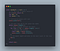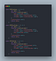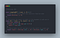How deep is your search? A brief look at DFS and BFS Search Algorithms

After you catch some pump-up vibes from Calvin harris or some very chill vibes from the Beegees, let’s take a stab at understanding ‘Depth-First Search’ and ‘Breadth-First Search’ traversals of trees.

Firstly you might be asking, why do I care? Well, a few reasons:

1. It may come up as a great solution to an algorithm for an interview question.
2. It is a great way to practice wrapping your head around good use cases for recursive functions.
3. There are practical use cases for games such as figuring out Chess moves in advance or calculating/finding choices one can make against an opponent.

So jumping into it now, let’s say you have a tree of nodes and you want to find a particular node on that tree. We will focus on Tree traversal vs graphs for these examples. There are two ways to traverse the tree and get information about the nodes. Breadth-First Search and Depth-First Search.

Breadth-First is a method of visiting each node layer by layer from the top to the bottom, parent to child to grand-children, and so forth.

A very basic example of this can be seen with this Leetcode problem. Let’s solve it.

559. Maximum Depth of N-ary Tree

‘Given an n-ary tree, find its maximum depth.

The maximum depth is the number of nodes along the longest path from the root node down to the farthest leaf node.’

` Input: root = [1,null,3,2,4,null,5,6] Output: 3`

The Problem: In this problem, it’s pretty self-explanatory, we want to count how deep this tree goes.

If you saw this in real life, on a tree and wanted to count how many branches from the leaf to the top of the tree, how would you do it? I think it is helpful to imagine a real-life scenario and how you would do it to derive your algorithm solution.

I would imagine visually, I would look for the longest branches to leaf furthest from the top of the tree and count from the leaf to the top. Which is pretty much how we are going to accomplish this. Except the problem is your computer doesn’t have eyes(or does it…) and can’t visually see the longest leaf. So we need to count the breadth of the tree to find out.

Breadth: The distance or measurement from side to side of something.

The solution: We need to search the tree through all its branches and then count branches' length to find the max branch length.

To implement this:

1. We will use a queue(FIFO) to store the node we visit, and a variable to count how deep the children/branches go.
2. Add the root node to the queue to create a starting point for our search.
3. Loop through the queue as long as the queue isn’t empty.
4. Then dequeue a node from the queue into a variable and add that nodes children to the queue
5. Once this is complete, we will increment our count by 1 before we get to the next loop. Which demonstrates the level of the tree we have traversed.Max Depth Solution

Alrighty, now let's move onto Depth-First Search.

Depth-First Search is the method by which you visit nodes vertically, before visiting sibling nodes. Whereas BFS you are traversing horizontally level by level, with DFS we are traversing the siblings or nodes to the side after we have visited the nodes down or vertically.

There are variations with DFS including Pre-Order, In-Order, and Post-Order. These change how you add the traversed nodes order in the queue as you visit each node.

Pre-Order: We visit the node first, and traverse all the nodes to the left of the and then all the nodes to the right.

Post-Order: We visit all children before visiting the root node. We traverse the left side all the way to the end and it to the queue and then back up the tree and all the way to the bottom right branch and then up the right.

In-Order: We visit the leftmost child, then its parent and if it has another child, we visit that node’s parents up to the root. Then we traverse the right in the same manner.

Here is some example code that expresses these rules.Udemy Data Structure and Algorithms course

Let’s take a look at an easy Leetcode problem to exemplify DFS and finish up this topic.

938. Range Sum of BST (Binary Search Tree)

Given the node of a binary search tree, return the sum of values of all nodes with a value in the range .

`Input: root = [10,5,15,3,7,null,18], low = 7, high = 15Output: 32`

The problem: This ask here is also quite self-explanatory, given the range (low ≤nodes ≤ high) we want to traverse the tree and find the sum of those node values. In this Input and Output example, we see nodes 7 + 10 + 15 which is 32.

The solution: Because this is a binary search tree, we have an order to the node values from the least value to the bottom left to the highest value on the bottom right. This order lends itself well for a DFS to find a value at the bottom left and traverse up and to the right, we can more easily identify node values within the high and low range.

To implement this:

1. We can create a function to recursively traverse all the nodes starting from the root, to the left of the root, and right of the root.
2. Within that function, as we traverse, if the value of the node is greater than the low and less than the high, we will add it to the value of a result.
3. When we have completed the recursive traversal of the tree, we will return the result.RangeSum Solution

There are a few ways one can accomplish this solution, but I found this example to be very cool and pushes you to learn an interesting recursive solution.

Please feel free to share any feedback and comment if I made any mistakes :). Hope this was helpful!

Padawan Software Engineer

## More from Jake Hyde

Padawan Software Engineer